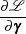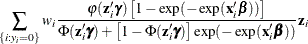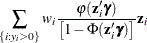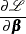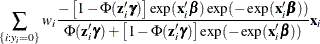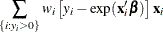Zero-Inflated Poisson Regression

In the zero-inflated Poisson (ZIP) regression model, the data generation process referred to earlier as Process 2 is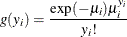where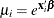. Thus the ZIP model is defined as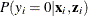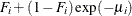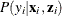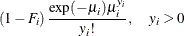The conditional expectation and conditional variance of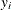are given by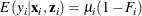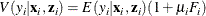Note that the ZIP model (as well as the ZINB model) exhibits overdispersion since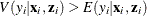.

In general, the log-likelihood function of the ZIP model is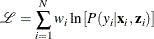After a specific link function (either logistic or standard normal) for the probability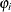is chosen, it is possible to write the exact expressions for the log-likelihood function and the gradient.

ZIP Model with Logistic Link Function

First, consider the ZIP model in which the probabilityis expressed with a logistic link function—namely,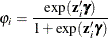The log-likelihood function is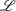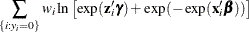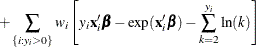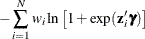See Poisson Regression for the definition of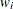.

The gradient for this model is given by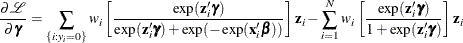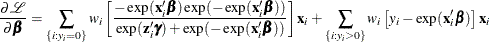ZIP Model with Standard Normal Link Function

Next, consider the ZIP model in which the probabilityis expressed with a standard normal link function: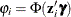. The log-likelihood function is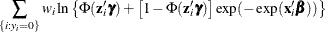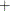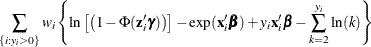See Poisson Regression for the definition of.

The gradient for this model is given by